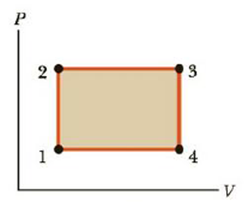Chapter 12, Problem 66AP

Chapter
Section
Textbook Problem

When a gas follows path 123 on the PV diagram in Figure P 12.66, 418 J of energy flows into the system by heat and −167J of work is done on the gas. (a) What is the change in the internal energy of the system? (b) How much energy Q flows into the system if the gas follows path 143? The work done on the gas along this path is −63.0 J. What net work would be done on or by the system if the system followed (c) path 12341 and (d) path 14321? (e) What is the change in internal energy of the system in the processes described in parts (c) and (d)?Figure P12.66

(a)

To determine
The change in internal energy of the system in the path 123.

Explanation

Given Info:

The energy flows in to the system by heat is 418J .

The work done on the gas is 167J .

Formula to calculate the change in internal energy of the system is,

ΔU13=W123+Q123

• W123 is the work done on the gas in path 123
• Q123 is the energy flows into the system by heat in the path 123

Substitute 418

(b)

To determine
the energy flows into the system if the gas follows the path 143.

(c)

To determine
The work done on or by the system if the system followed the path 12341.

(d)

To determine
The work done on or by the system if the system followed the path 14321.

(e)

To determine
The change in internal energy of the system in the process described in (c) and (d).

Still sussing out bartleby?

Check out a sample textbook solution.

See a sample solution

The Solution to Your Study Problems

Bartleby provides explanations to thousands of textbook problems written by our experts, many with advanced degrees!

Get Started

Over-the-counter drugs for obesity are most often effective and pose little risk.

Nutrition: Concepts and Controversies - Standalone book (MindTap Course List)

(Chemical Connections 26G) What is the epigenome?

Introduction to General, Organic and Biochemistry

What is a life cycle?

Biology: The Dynamic Science (MindTap Course List)